# A transmission tower is held by three guy wires attached to a pin at A and anchored by bolts at B, C, and D . If the tension in wire AC is 590 lb, determine the vertical force P exerted by the tower on the pin at A

Question-AnswerCategory: Engineering MechanicsA transmission tower is held by three guy wires attached to a pin at A and anchored by bolts at B, C, and D . If the tension in wire AC is 590 lb, determine the vertical force P exerted by the tower on the pin at A

### A transmission tower is held by three guy wires attached to a pin at A and anchored by bolts at B, C, and D . If the tension in wire AC is 590 lb, determine the vertical force P exerted by the tower on the pin at A.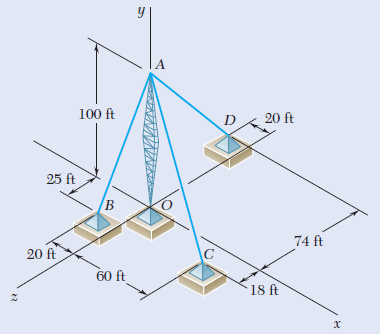Step 1

we solve this question by resolution of component of force in 3D ( 3 dimensions).
given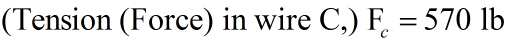dimensions of all 3 wires from the origin O is given.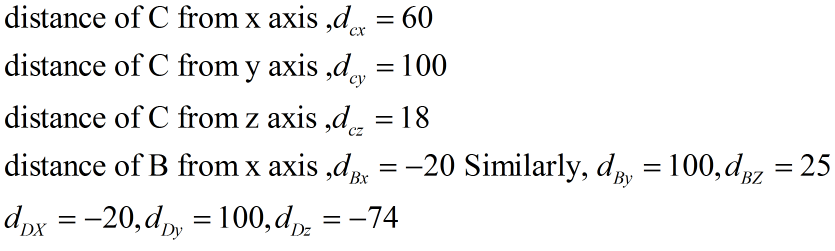Step 2Similarly dB=105 ft; dD=126 ft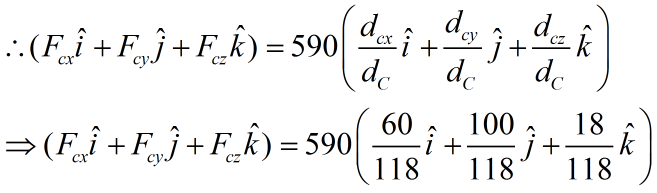Similarly, for pin at point Band
For pin at point D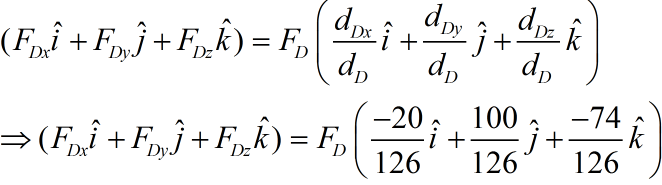Boundary Condition- Resultant Sum of force in x and y direction is 0
this above constraint is used to find FB and FD
(60/118)590+(-20/105)FB+(-20/126)FD=0Â Â
& (18/118)590+(25/105)FB+(-74/126)FD=0
i.e (20/105)FB+(20/126)FD=(60/118)590
& (25/105)FB+(-74/126)FD=-(18/118)590
solving above two equations we get
FB=1081.82 lb
FD=591.82 lb

Step 3

we need to find vertical force P exerted by the tower on the pin at A
=Fcy+FBY+FDY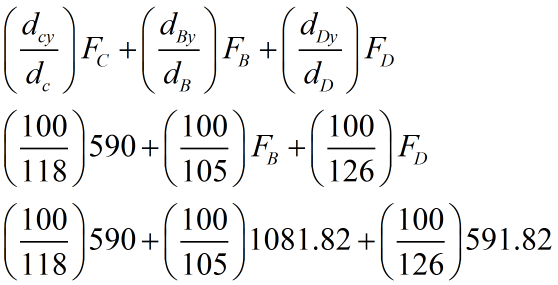=2000 lb